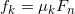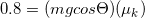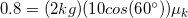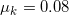# AP Physics B : Understanding Friction

## Example Questions

### Example Question #13 : Ap Physics 1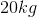crate is sitting on a rough floor with coefficient of static friction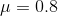. A man tries to push the crate horizontally. What force must the man exert on the crate in order to start moving the crate?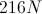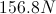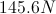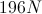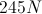Explanation:

We know that normal force on a flat surface is equal and opposite the force of gravity.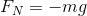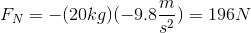Then, find the friction force between the crate and the floor using the equation: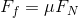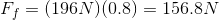This force is the minimum force required to start moving the crate.

### Example Question #7 : Force Of Friction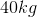box is initially sitting at rest on a horizontal floor with a coefficient of static friction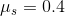. A horizontal pushing force is applied to the box. What is the maximum pushing force that can be applied without moving the box?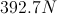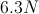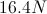Explanation:

The maximum force that can be applied will be equal to the maximum value of the static friction force. The formula for friction is: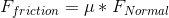We also know that the normal force is equal and opposite the force of gravity.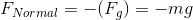Substituting to the original equation, we can rewrite the force of friction.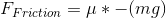Using the given values for the coefficient of friction and mass, we can calculate the force using the acceleration of gravity.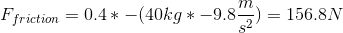### Example Question #4 : Force Of Friction

A man pulls a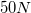box up a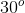incline to rest at a height of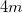. He exerts a total of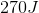of work. What is the coefficient of friction on the incline?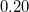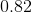We must know the mass of the box to solve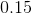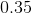Explanation:

Work is equal to the change in energy of the system. We are given the weight of the box and the vertical displacement, which will allow us to calculate the change in potential energy. This will be the total work required to move the box against gravity.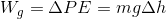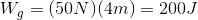The remaining work that the man exerts must have been used to counter the force of friction acting against his motion.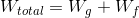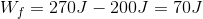Now we know the work performed by friction. Using this value, we can work to solve for the force of friction and the coefficient of friction. First, we will need to use a second formula for work: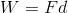In this case, the distance will be the distance traveled along the surface of the incline. We can solve for this distance using trigonometry.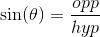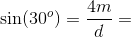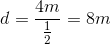We know the work done by friction and the distance traveled along the incline, allowing us to solve for the force of friction.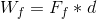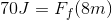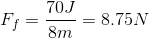Finally, use the formula for frictional force to solve for the coefficient of friction. Keep in mind that the force on the box due to gravity will be equal to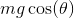.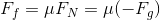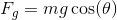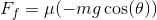Plug in our final values and solve for the coefficient of friction.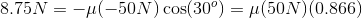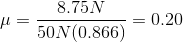### Example Question #1 : Force Of Friction

A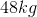crate is loaded onto a pick-up truck, and the truck speeds away without the crate sliding. If the coefficient of static friction between the truck and the crate is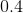, what is the maximum acceleration that the truck can undergo without the crate slipping?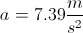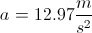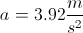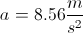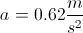Explanation:

In order for the crate to not slide, the truck has to exert a frictional force on it. The force of friction is related to the normal force by the coefficient of friction.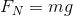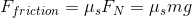This frictional force comes from the acceleration of the truck, based on Newton's second law.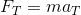The two forces will be equal when the truck is at maximum acceleration without the crate moving.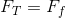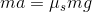Solve for the acceleration.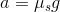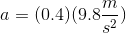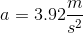### Example Question #41 : Newtonian Mechanics

A 2kg box is at the top of a ramp at an angle of 60o. The top of the ramp is 30m above the ground. The box is sitting still while at the top of the ramp, and is then released.

Imagine that the net force on the box is 16.5N when sliding down the ramp. What is the coefficient of kinetic friction for the box?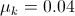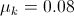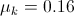The kinetic coefficient of friction cannot be determined while the box is movingExplanation:

Since the box is moving when the net force on the box is determined, we can calculate the coefficient of kinetic friction for the box. The first step is determining what the net force on the box would be in the absence of friction. The net force on the box is given by the equation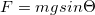.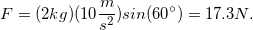The difference between the frictionless net force and the net force with friction is 0.8N. This means that the force of kinetic friction on the box is 0.8N, acting opposite the direction of motion. Knowing this, we can solve for the coefficient of kinetic friction using the equation